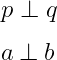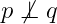# How to denote perpendicular symbol(⟂) in LaTeX?

If two straight lines are at an angle of 90° to each other, then both straight lines are perpendicular to each other. This perpendicular symbol consists of a horizontal bar and a vertical bar.

 Properties Value Symbol Perpendicular Package Default Command \perp Argument No Example \perp → ⊥
\documentclass{article}
\begin{document}
$p \perp q$
$a \: \bot \: b$
\end{document}

Output :If you look at the program above, you can see that the \prep command is used to represent perpendicular symbols. And \: has been used for space.

Many times, not perpendicular symbols are used, in which case the \not\prep command is used.

\documentclass{article}
\begin{document}
$p \not\perp q$
\end{document}

Output :#### Md Jidan Mondal

LaTeX expert with over 10 years of experience in document preparation and typesetting. Specializes in creating professional documents, reports, and presentations using LaTeX.